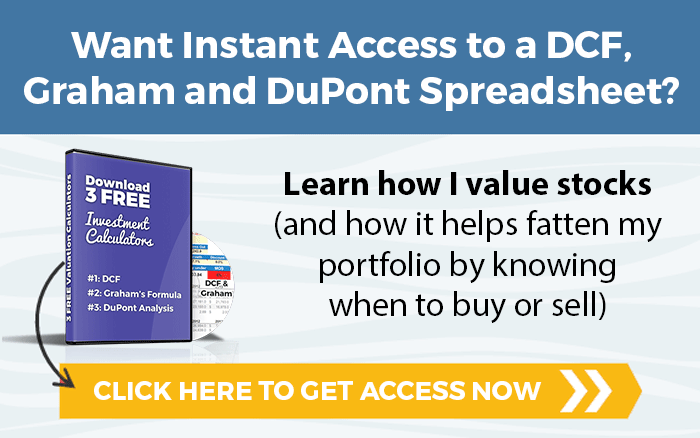# How to Calculate Your Time Weighted Return Portfolio PerformanceWritten by

Jae Jun

What You’ll Learn

• Why you should use Time Weighted Returns
• Portfolio performance examples
• 2 different methods we use

To get this kind of information and other exclusive articles before regular readers, get on the VIP Mailing List today.

********

In its most basic form, the objective of tracking performance is to see whether your portfolio is growing.

However, portfolio performance should also provide insight into the performance of the portfolio manager in how well securities are being selected and allocated. Whether you are selecting stocks yourself, or you have a person managing your money, measuring and keeping a yard stick is important.

There are many ways to calculate performance – the simplest method being a simple percentage change calculation of the ending and beginning value.

If you use any sort of free portfolio tracking tool, this is most likely method used.

However, due to cash flows in and out of a portfolio that affect portfolio values and return calculations, we use the Time Weighted Return for our portfolio calculations in the OSV Online app.

## Why Time Weighted Returns to Measure Performance?

The Time Weighted Return calculates performance based strictly on the manager’s actions.

It “ignores” the cash in and out.

If you start with \$100, do nothing but deposit \$100, the ending value will be \$200. Does this mean your portfolio went up 100%?

No.

The beauty of the Time Weighted Return is that it only factors in the portfolio manager’s actions by breaking up the overall period into subperiods and then linking each subperiod to get the total time weighted return.

These subperiods are linked together (compounded) to calculate the total return for the overall period.

In order to calculate performance as accurately as possible, the time weighted subperiods are calculated at the end of each trading day.

Don’t worry I have an example to show you step by step.

We use the same approach and calculation as Interactive Brokers. Buys and sells are accounted for in the same day. The full paper to Interactive Brokers white paper is linked in the “Resources” section.

## Formula for Time Weighted Returns

The first part of calculating the subperiod return is:where:

• RN = Subperiod Return
• EMV = Ending Market Value
• BMV = Beginning Market Value
• CF = Cash Flow

Each subperiod return is then linked with the following formula to get the total return.

TWR = [(1 + RN) × (1 + RN) × … − 1] × 100

## Time Weighted Return Example

Example of subperiod returns throughout January.The subperiod returns are then linked to calculate the total weighted return.

TWR = [(1 + RN) × (1 + RN) × … − 1] × 100

[(1 + 1.15%) × (1 +− 2.47%) × (1 + 1.22%) × (1 + 16.86%) × (1 + 4.7%) − 1] × 100 = 22.19%

## Equal Weighted Portfolio Performance

Within the portfolio performance section of Old School Value, you will see two sets of numbers.

1. Total Portfolio Performance & Total Real Value Equal
2. Weighted Portfolio Performance & Total Equal Weighted Value

## Total Portfolio Performance & Total Real Value

The chart and calculations for Total Portfolio Performance and Total Real Value calculates the return based on your actual portfolio weightings.

It tracks how the actual value of the portfolio is growing.

In this purely theoretical and random example, the starting value of the portfolio was \$3,600 but grew to \$15,700 after cash flows in and out.## Equal Weighted Portfolio Performance & Total Equal Weighted Value

The Equal Weighted calculation takes the value of the portfolio, the stocks you hold, the number of shares and creates an equally weighted portfolio with \$100,000.

In this way, it provides a clearer picture of how well the portfolio manager selects securities.

A highly concentrated or heavily weighted portfolio is a great way to beat the market, but what if one stock drove the portfolio value while the remaining 9/10 declined in value?

What does that tell you about the likelihood of future performance?

This chart is based on the exact same portfolio as above, but it is equally weighted across a beginning \$100,000 value.The ending value of the portfolio is \$422,800.

Again in this theoretical example, the portfolio manager did a majestic job of picking successful stocks and creating value.

For more reading and extra information on calculating portfolio returns and time weighted returns, read the links below.

To play with a live working demo and see the portfolio calculations in action, you can visit the OSV Portfolio section.

## Resources

To get this kind of information and other exclusive articles before regular readers, get on the VIP Mailing List today.

********

### What is Old School Value?

Old School Value is a suite of value investing tools designed to fatten your portfolio by identifying what stocks to buy and sell.

It is a stock grader, value screener, and valuation tools for the busy investor designed to help you pick stocks 4x faster.

Check out the live preview of AMZN, MSFT, BAC, AAPL and FB.